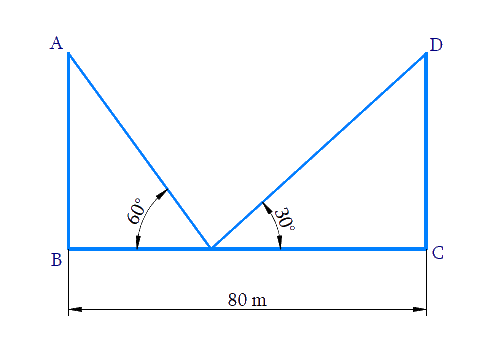# Ex.9.1 Q10 Some Applications of Trigonometry Solution - NCERT Maths Class 10

Go back to  'Ex.9.1'

## Question

Two poles of equal heights are standing opposite each other on either side of the road, which is $$80\,\rm{m}$$ wide. From a point between them on the road, the angles of elevation of the top of the poles are $$60^\circ$$ and $$30^\circ$$ respectively. Find the height of the poles and the distances of the point from the poles.

Video Solution
Some Applications Of Trigonometry
Ex 9.1 | Question 10

## Text Solution

What is Known?

(i) The poles of equal height.

(ii) Distance between poles $$= 80\,\rm{m}$$

(iii) From a point between the poles, the angle of elevation of the top of the poles are $$60^\circ$$ and $$30^\circ$$ respectively.

#### What is Unknown?

Height of the poles and the distances of the point from the poles.Reasoning:

Let us consider the two poles of equal heights as $$AB$$ and $$DC$$ and the distance between the poles as $$BC$$. From a point $$O$$, between the poles on the road, the angle of elevation of the top of the poles $$AB$$ and $$CD$$ are $$60^\circ$$ and $$30^\circ$$ respectively.

Trigonometric ratio involving angles, distance between poles and heights of poles is $$\tan \theta$$

Steps:

Let the height of the poles be $$x$$

Therefore $$AB=DC=x$$

In $$\Delta AOB,$$

\begin{align}\text{tan 6}{{\text{0}}^{0}}&=\frac{AB}{BO} \\ \sqrt{3}&=\frac{x}{BO} \\ BO&=\frac{x}{\sqrt{3}}\quad\dots\left( \text{i} \right) \end{align}

In $$\Delta OCD,$$

\begin{align}& \tan \text{ }{{30}^{0}}=\frac{DC}{OC} \\& \frac{1}{\sqrt{3}}=\frac{x}{BC-BO} \\ & \frac{1}{\sqrt{3}}=\frac{x}{80-\frac{x}{\sqrt{3}}}\qquad\left[ \text{from }\left( \text{i} \right) \right] \\ & 80-\frac{x}{\sqrt{3}}=\sqrt{3}x \\ & \frac{x}{\sqrt{3}}+\sqrt{3}x=80 \\ & x\left( \frac{1}{\sqrt{3}}+\sqrt{3} \right)=80 \\ & x\left( \frac{1+3}{\sqrt{3}} \right)=80 \\ & x\left( \frac{4}{\sqrt{3}} \right)=80 \\ & x=\frac{80\sqrt{3}}{4} \\ & x=20\sqrt{3} \\ \end{align}

Height of the pole $$x = 20\sqrt 3 {\rm{ }}\,\rm m$$

Distance of point from $$O$$ the poles $$AB$$

\begin{align} BO&=\frac{x}{\sqrt{3}} \\ & =\frac{20\sqrt{3}}{\sqrt{3}} \\ & =20 \end{align}

Distance of point from $$O$$ the poles $$CD$$

\begin{align}OC&=BC-BO \\ & =80-20 \\ & =60 \end{align}

Height of the poles are  $$20\sqrt{3}\text{ }\rm{m}$$  and the distances of the point from the poles are $$20\rm{m}\text{ and }60\rm{m}$$ .

Learn from the best math teachers and top your exams

• Live one on one classroom and doubt clearing
• Practice worksheets in and after class for conceptual clarity
• Personalized curriculum to keep up with school